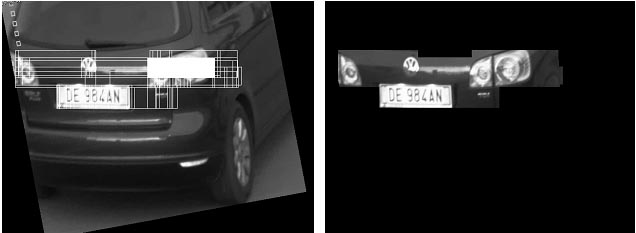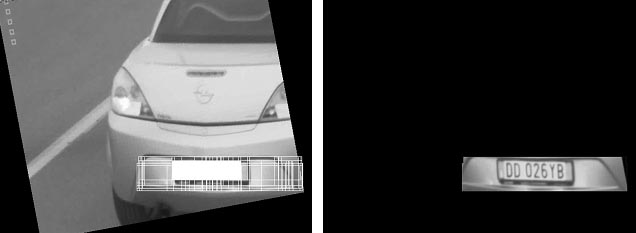# Plate detection algorithm

## Part 1: project profile

### Project name

Plate detection algorithm

### Project short description

The aim of the project is to merge two plate detection algorithms previously developed trying to make the best of them. Different approaches were studied underlying pro and con.

### Dates

Start date: 2008/02/01

End date: 2008/05/19

### People involved

##### Students who worked on the project in the past

Andrea Machina - as a project for courses Image Analysis and Synthesis, prof. Caglioti, and Laboratorio di Intelligenza Artificiale e Robotica, prof. Lanzi

## Part 2: project description

### The Problem

Dealing with plate detection problems two algorithms were developed based on different characteristics.

The first one is based on the application of different masks to the original image. Each mask puts in evidence a characteristic of a plate: black stripes on white background, the rectangular shape etc. In the end a procedure merges all the images previously calculated achieving the result image where the plate is put in evidence.

The second algorithm is based on the research of vertical and horizontal lines looking for the ones that, intersecting with the others, can describe the shape of a plate maintaining its proportions.

The first algorithm is quite heavy but accurate, the second one is faster but not robust. The aim of this project is to merge these algorithms trying to make the best of them.

### Preliminary studies

This project describes four possible solutions.

1) The second algorithm (the faster one) is applied to the original image performing a first selection of region of interest based on geometrical characteristics. Each candidate found with this algorithm has been used to build a new binary mask (a selection mask), with the dimensions of the original image, where, if a pixel (x, y) is part of a candidate the corresponding element of the mask (x, y) is put to 1, 0 otherwise. The first algorithm is then applied to the original image but, during the merging mask is considered also the new selection mask. In this way we can reduce the amount of false positives.On the left: candidates found by the second algorithm. On the right: the selection mask calculated.On the left: candidates found by the second algorithm. On the right: the selection mask calculated.

2) As in the first solution the second algorithm is applied. The first algorithm is then applied not on the whole original image but on each candidate found by the second algorithm. This solution aims to reduce the first algorithm complexity applying it only on the selected candidates.

3) This approach uses the second algorithm to find all the candidates. The first algorithm is then applied and the medium value is calculated on each candidate found. Then they are ordered using the value found. The first element of the list in this approach is an element with the photometric characteristics of a plate and with the best medium value calculated with the first algorithm.

4) This approach is a consideration for future applications, it hasn’t been implemented in this project. It is based on the recognition of characters in the candidates. In this approach the second algorithm is applied because it’s the faster, the best solution returned is then analyzed by the OCR algorithm. If the OCR can find in that area a determined number of characters this candidate can be considered the plate we were looking for. Otherwise the first algorithm is applied looking for a best solution. The OCR is then applied on the candidate returned by the first algorithm.

### Results and problems

The first three algorithms were implemented in this project and tested on a set of images giving satisfactory results on the whole set only using the first approach. The second algorithm gave good results only on some images.

1) The first approach is the one that gave the best results, as we can see in the images the candidates in the end of the algorithm are reduced by the second algorithm and, in some images where the second algorithm returned the wrong candidate, applying the first algorithm gave the right result.

2) This approach gave good results only in few cases. The idea was to reduce the area analyzed by the first algorithm but the second algorithm applied on some images returned a lot of candidates, the sum of the pixels of the whole candidates found was bigger than the number of pixels in the original image.

3) This solution didn’t gave good results because it is heavy influenced by noises. Small white areas in candidates can in fact change the order of the elements in the list influencing the medium value calculated.